Start typing, then use the up and down arrows to select an option from the list.Jules Bruno
148views
1
6
in this example of states. What is the Moller soluble ity of iron three hydroxide in a solution that is buffered at Ph 3.50 at 25 degrees Celsius. Here they also tell us that our K S P, which is R Saudi ability product constant, is equal to 6.3 times 10 to the negative 38 at 25 degrees Celsius. All right, so they tell us k sp. So that means we're dealing with an ionic compound in in solid form. And we're talking about how it breaks up into its ions when dropped into solution. So this breaks up in tow. Iron three ion and there are three hydroxide ions. So remember, we're dealing with K sp. We do a nice chart in a nice chart. We ignore solids and liquids. And if something has a K S P value, it will be an ionic solid. So here my reacted as an X through it. Now here's the thing. This is being thrown into solution that is at a ph of 3. But remember, since it's a metal with an O. H group, this represents a base bases don't deal with pH. They deal with P o H. So how do we figure out p o h? Remember P O. H will equal 14 minus pH. And why? That's because ph plus p o H together gives us 14. So it's 14 minus 3.50 which means our p O. H is 10.50. Now, if we know our p o h, that means that we know what our O. H minus concentration will be. Remember, our next formula is o h minus equals 10 to the negative p o. H. So plugging the 10.50 that we just got So we do that it gives me 3. times 10 to the negative 11 Moeller. So that's the concentration of our hydroxide. Um solution now realize that we're taking this ionic compound and we're dumping it in a solution that has a concentration of 3.16 times 10 to the negative Mueller hydroxide ion realize that our solution is made up of this ion. But our ionic compound that's breaking up also has the same exact ion. We're going to stay here that since both of these ions are the same. We call this the common ion effect. So that would mean that this is also 3.16 times 10 to the negative 11. So this is a common I, in effect question just phrased in a different way. We don't have any iron three initially, so that zero So this is plus X plus three x plus x and then bring down everything 3. times 10 to the negative, 11 plus three X Now remember, K SP just equals products here because are reacting is a solid, so we're going to ignore it. So here K s P equals F E three plus times O h minus. Remember, the coefficient here is going to become a power. So this is to the third power now K SP here is 6.3 times 10 to the negative 38 at equilibrium. I am three equals x And remember, if we have actual numbers in front of our X variable, that means we can ignore our ex variables. All of this is ignored, so that will be 3.16 times 10 to the negative 11. Cute. So we divide both sides by 3.16 times 10 to the negative cube. Okay, so we divide them by that. So we do that we're going to get as our answer for X X equals 2.0 times 10 to the negative. Six. Mueller. Now we're looking for the Mueller saw the ability of our Ionic compound and any time we're looking for the suitability of the Ionic compound, that's always just equal to X. So this would be our final answer. This would represent the concentration or Mueller Sigh ability off iron three hydroxide.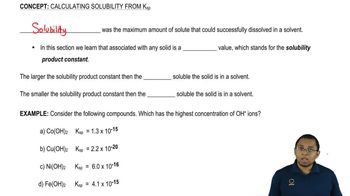01:30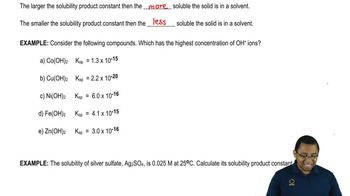01:5905:51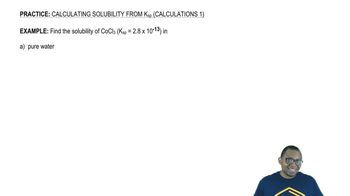05:21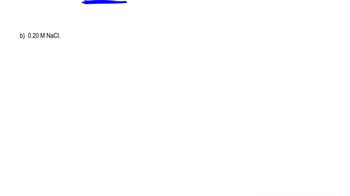04:11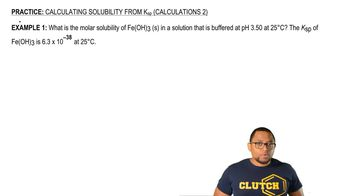04:5005:15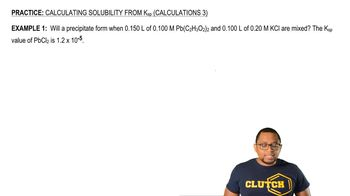07:1606:0503:47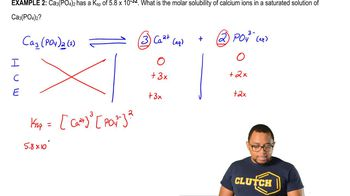04:1003:44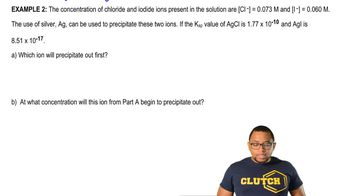03:36